Ex 16.2

Chapter 16 Class 11 Probability (Term 2)
Serial order wise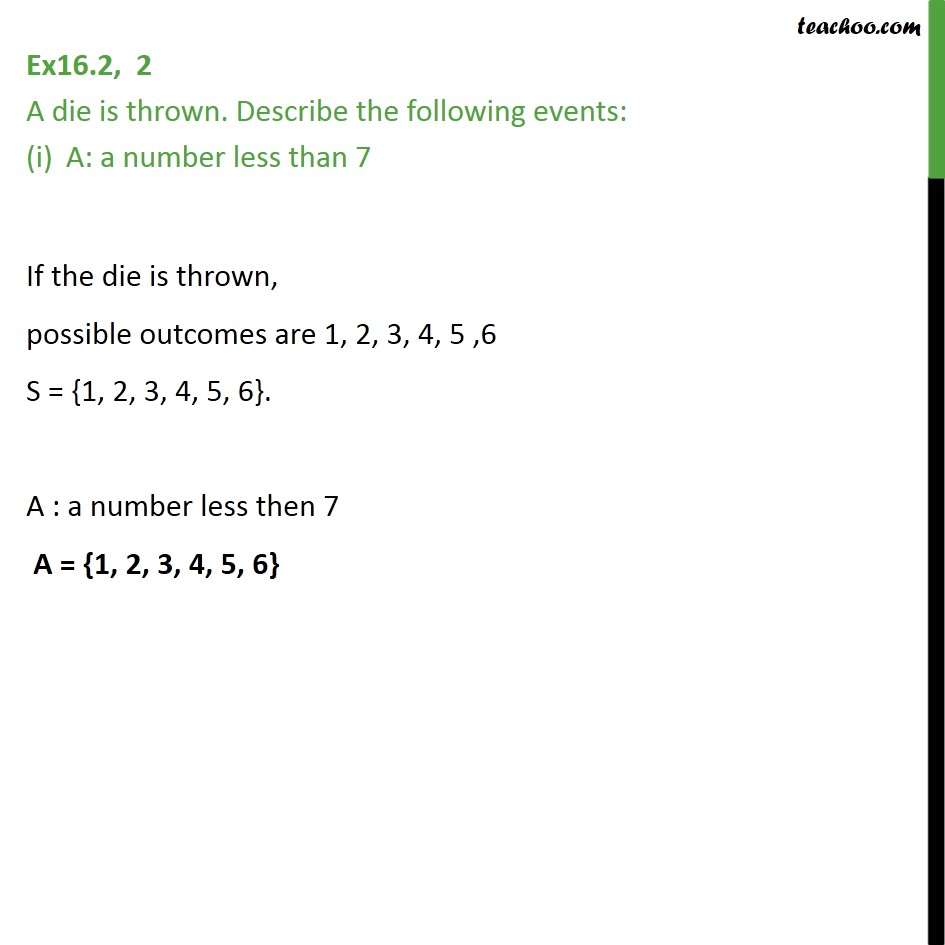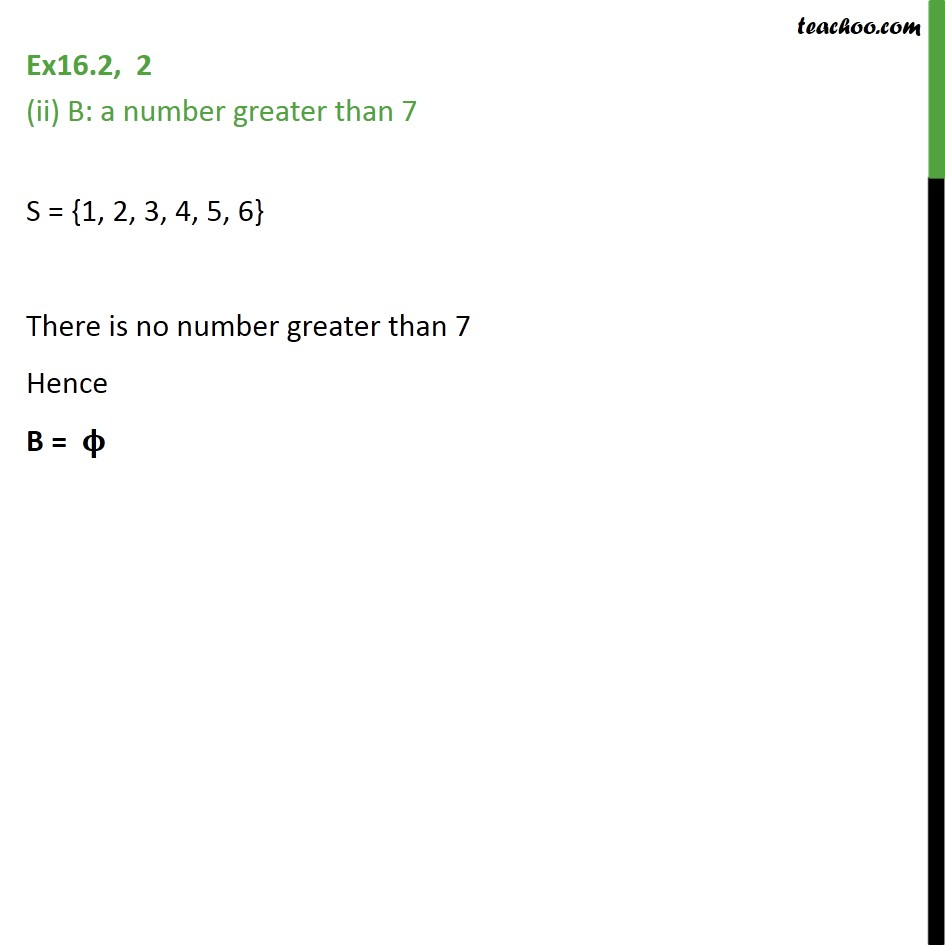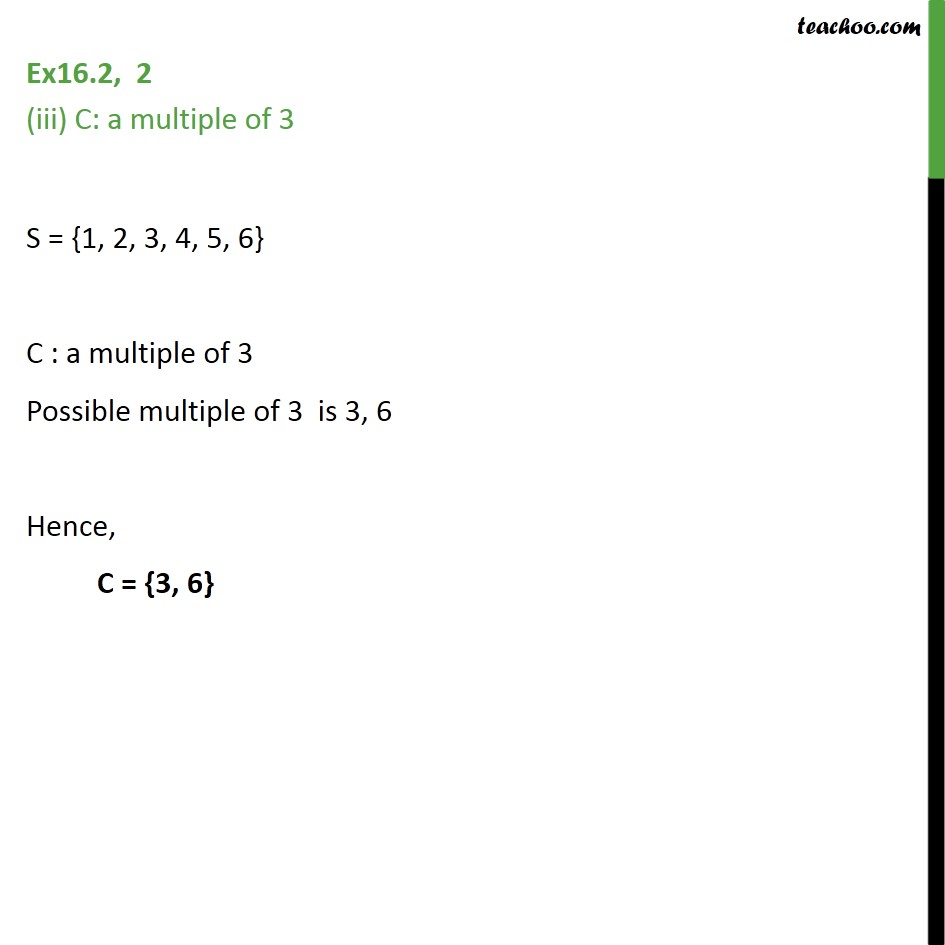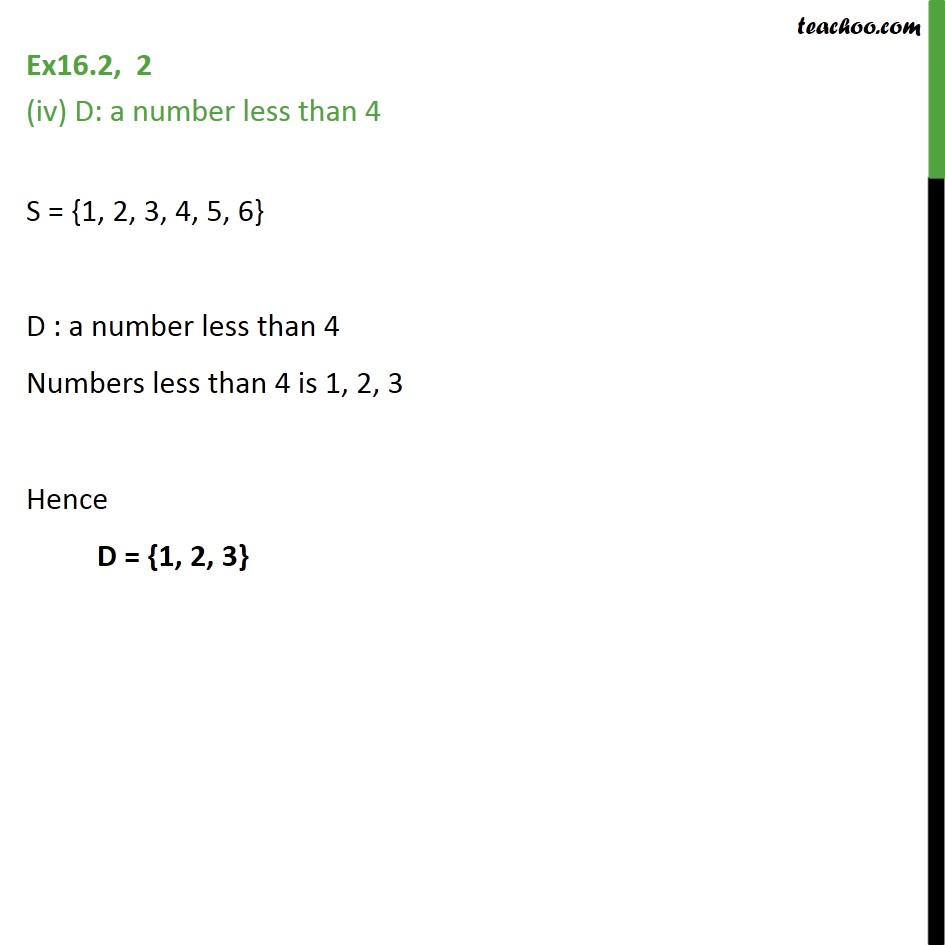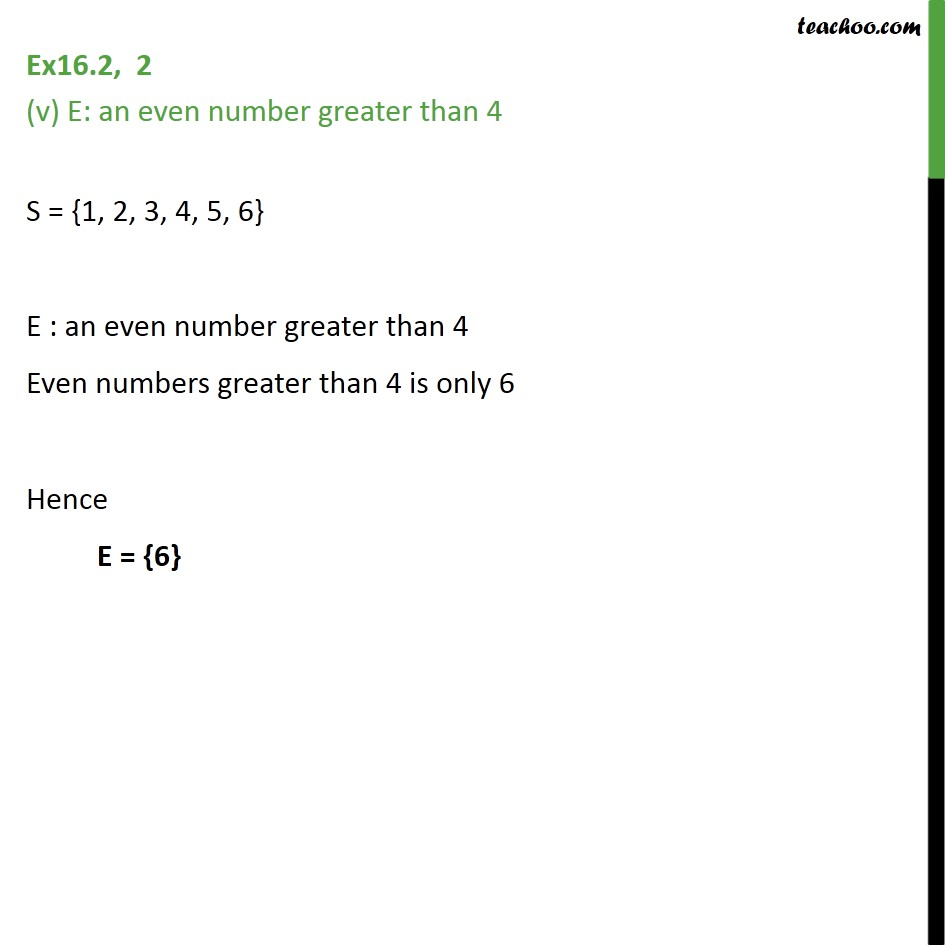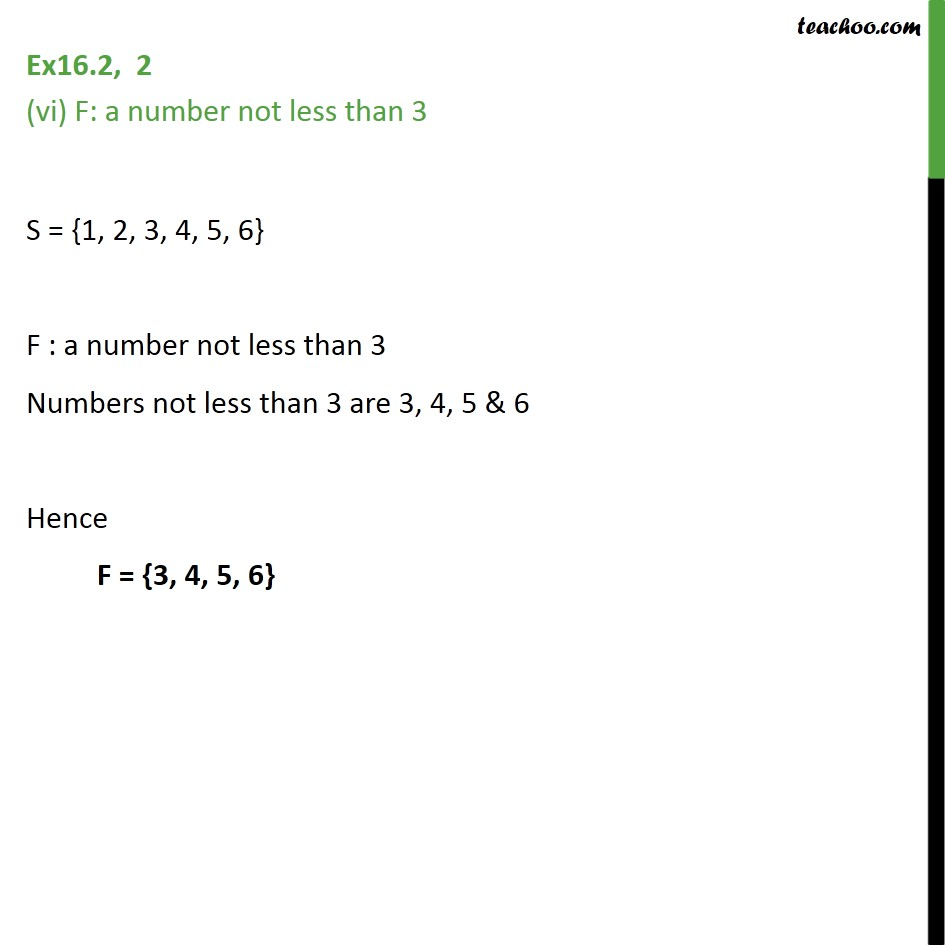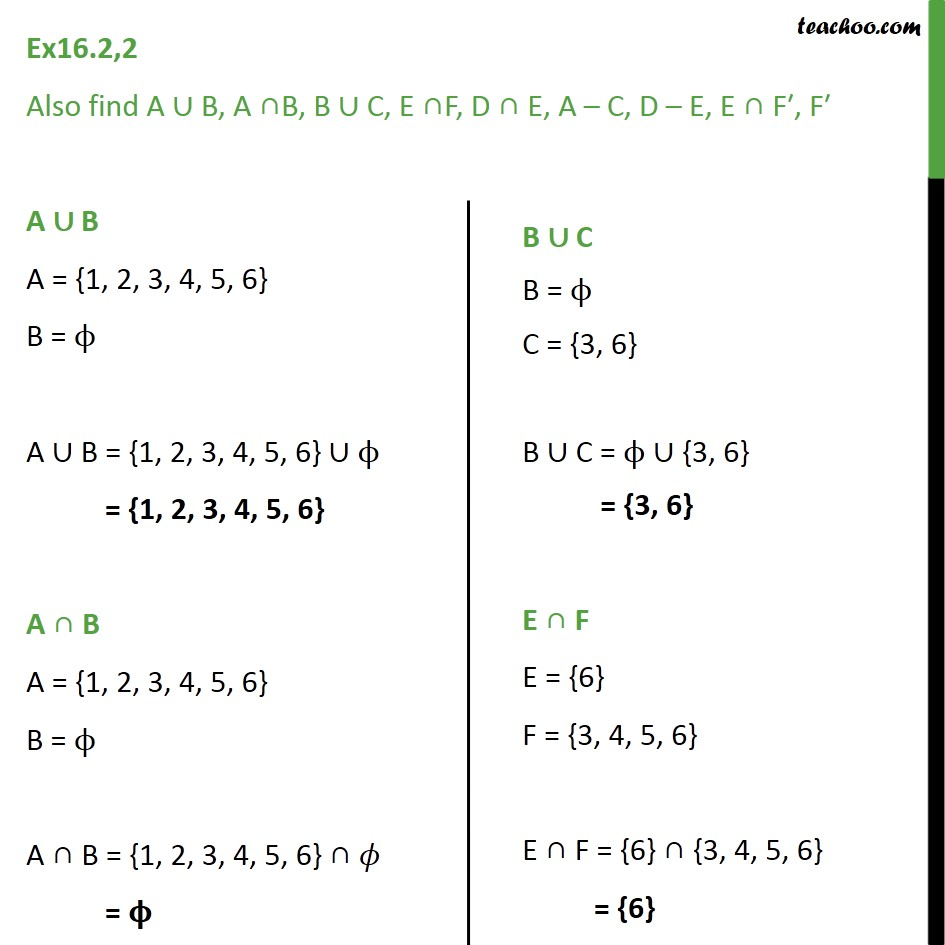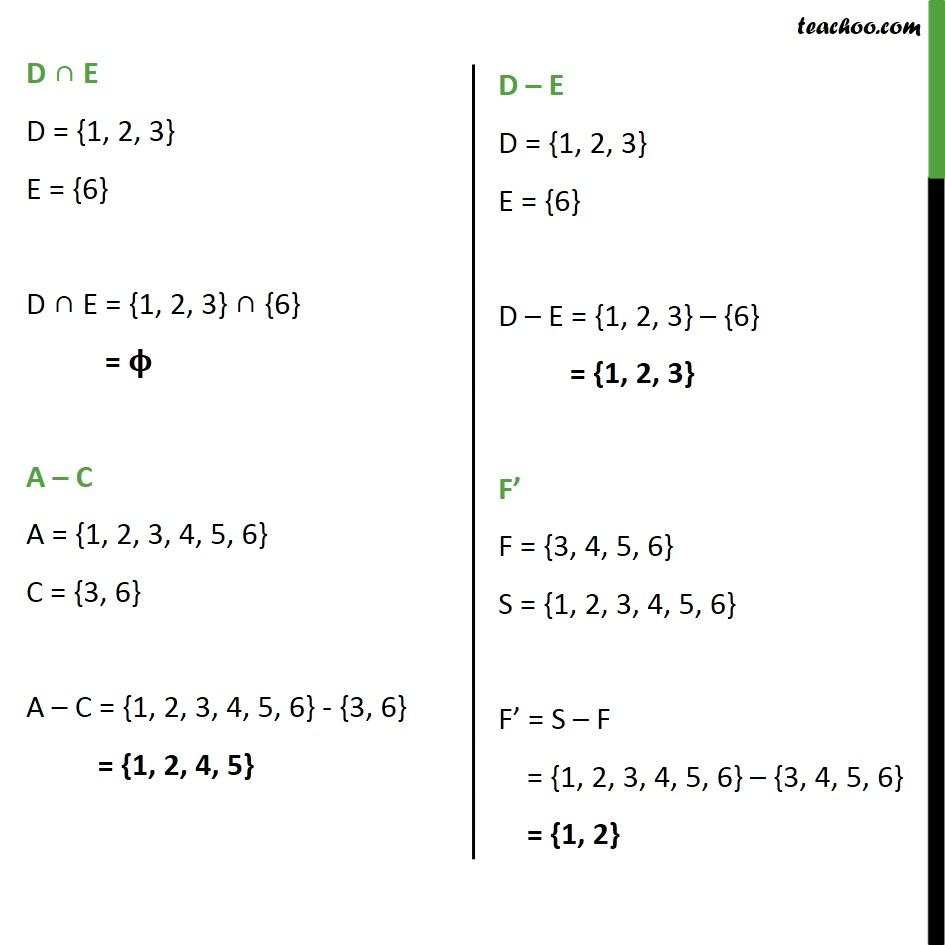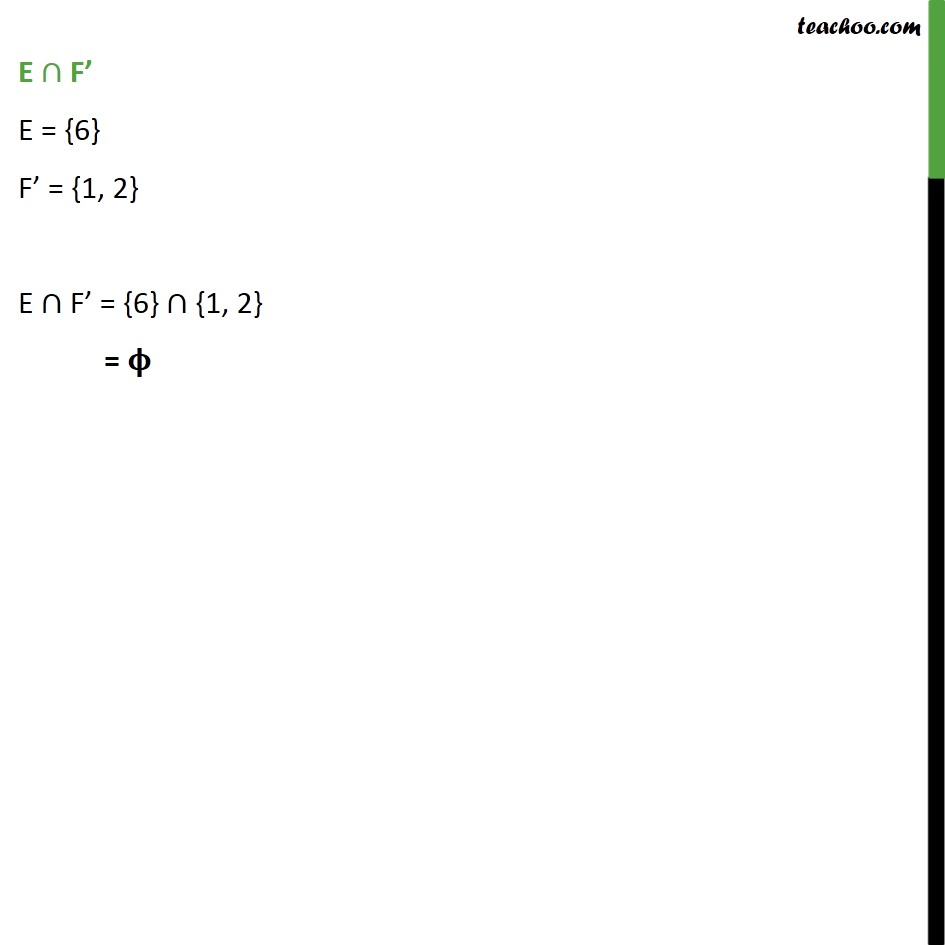641 students have Teachoo Black. What are you waiting for?

### Transcript

Ex16.2, 2 A die is thrown. Describe the following events: A: a number less than 7 If the die is thrown, possible outcomes are 1, 2, 3, 4, 5 ,6 S = {1, 2, 3, 4, 5, 6}. A : a number less then 7 A = {1, 2, 3, 4, 5, 6} Ex16.2, 2 (ii) B: a number greater than 7 S = {1, 2, 3, 4, 5, 6} There is no number greater than 7 Hence B = Ex16.2, 2 (iii) C: a multiple of 3 S = {1, 2, 3, 4, 5, 6} C : a multiple of 3 Possible multiple of 3 is 3, 6 Hence, C = {3, 6} Ex16.2, 2 (iv) D: a number less than 4 S = {1, 2, 3, 4, 5, 6} D : a number less than 4 Numbers less than 4 is 1, 2, 3 Hence D = {1, 2, 3} Ex16.2, 2 (v) E: an even number greater than 4 S = {1, 2, 3, 4, 5, 6} E : an even number greater than 4 Even numbers greater than 4 is only 6 Hence E = {6} Ex16.2, 2 (vi) F: a number not less than 3 S = {1, 2, 3, 4, 5, 6} F : a number not less than 3 Numbers not less than 3 are 3, 4, 5 & 6 Hence F = {3, 4, 5, 6} Ex16.2,2 Also find A B, A B, B C, E F, D E, A C, D E, E F , F A B A = {1, 2, 3, 4, 5, 6} B = A B = {1, 2, 3, 4, 5, 6} = {1, 2, 3, 4, 5, 6} A B A = {1, 2, 3, 4, 5, 6} B = A B = {1, 2, 3, 4, 5, 6} = D E D = {1, 2, 3} E = {6} D E = {1, 2, 3} {6} = A C A = {1, 2, 3, 4, 5, 6} C = {3, 6} A C = {1, 2, 3, 4, 5, 6} - {3, 6} = {1, 2, 4, 5} E F E = {6} F = {1, 2} E F = {6} {1, 2} =